How Cheenta works to ensure student success?
Explore the Back-Story

# Largest Common Divisor | PRMO-2014 | Problem 11Try this beautiful problem from Algebra based on Largest Common Divisor .

## Largest Common Divisor | PRMO | Problem 11

For natural numbers x$x$ and y$y$,let (x,y)$(x,y)$ denote the largest common divisor of x$x$ and y$y$. How many pairs of natural numbers x$x$ and y$y$ with xy$x\leq y$ satisfy the equation xy=x+y+(x,y)$xy=x+y+(x,y)$?

••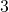•Answer:PRMO-2018, Problem 21

Pre College Mathematics

## Try with Hints

At first we have to find out the divisors that satisfy the equation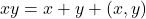.so we assume that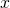=ak and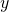=bk and try to find out the divisors

Can you now finish the problem ....

Let=ak and=bk, then (x,y)=k and (a,b)=1

ThereforeCan you finish the problem...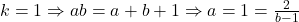For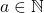, then (b-1) divides 2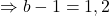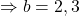Therefore a=1+2=3 and a=1+1=2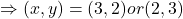Now for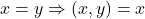so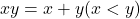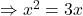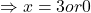Therefore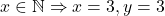ThereforeHence total number of pairs =3

## Subscribe to Cheenta at Youtube

Try this beautiful problem from Algebra based on Largest Common Divisor .

## Largest Common Divisor | PRMO | Problem 11

For natural numbers x$x$ and y$y$,let (x,y)$(x,y)$ denote the largest common divisor of x$x$ and y$y$. How many pairs of natural numbers x$x$ and y$y$ with xy$x\leq y$ satisfy the equation xy=x+y+(x,y)$xy=x+y+(x,y)$?

•••Answer:PRMO-2018, Problem 21

Pre College Mathematics

## Try with Hints

At first we have to find out the divisors that satisfy the equation.so we assume that=ak and=bk and try to find out the divisors

Can you now finish the problem ....

Let=ak and=bk, then (x,y)=k and (a,b)=1

ThereforeCan you finish the problem...For, then (b-1) divides 2Therefore a=1+2=3 and a=1+1=2Now forsoThereforeThereforeHence total number of pairs =3

## Subscribe to Cheenta at Youtube

This site uses Akismet to reduce spam. Learn how your comment data is processed.

### Knowledge Partner